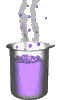Science class online
Chemistry and physics lessons

# Mechanics

Free online mechanics lesson for elementary school,  middle school and high school.Science class Chemistry Electricity Optics Mechanics Mechanics lessons Gravity - The solar system - motions of celestial bodies - Physical interactions - Effects of gravity - Weight of an object - Difference between weight and mass - Relationship between weight and mass  Energy - Energy basics - Potential energy - Kinetic energy - Mechanical energy - Translational Kinetic Energy - kinetic energy mass and speed

 _____________________________________________________     _____________________________________________________

## Gravity

### Difference between mass and weight

 1) Mass First, one of known properties of mass is its unit: mass is expressed in gram or in units derived from gram ( milligram, centigram etc ) In general mass is denoted m. Mass reflects the amount of matter which an object is composed. Thus mass depends on the number and kind of atoms in that object. Referring to definition of mass, if an object's composition isn't modified, it keeps the same mass. Particularly, the mass do not depend on place an object is. Wherever an object is ( On earth, on the Moon, on another planet and even in space ) its mass remain the same. ______________________________________ ______________________________________ 2) Weight Weight is the gravitational action exerted by Earth upon an object: it therefore depends on the same factors as gravity. - Weight depends on distance between an object and Earth: It lowers with height. - Weight depends on the celestial body's mass that exerts gravitational action: the more massive a celestial body is, the higher weight of an objetc is. Thus, weight is lower on The Moon than on Earth and far from all celestial bodies an object doesn't have weight   The weight of an object may vary while its mass remains constant. ______________________________________ ______________________________________
____________________________________

____________________________________